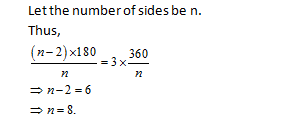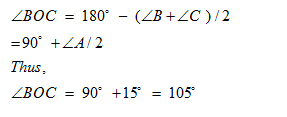• This is an assessment test.
• These tests focus on geometry and mensuration and are meant to indicate your preparation level for the subject.
• Kindly take the tests in this series with a pre-defined schedule.

## Geometry and Mensuration: Test 12

Congratulations - you have completed Geometry and Mensuration: Test 12. You scored %%SCORE%% out of %%TOTAL%%. You correct answer percentage: %%PERCENTAGE%% . Your performance has been rated as %%RATING%%
 Question 1
A man standing in one corner of a square football field observes that the angle subtended by a pole in the corner just diagonally opposite to this corner is 60o. When he retires 80 m from the corner, along the same straight line, he finds the angle to be 30o. The length of the field, in m, is:
 A 40 B 20√2 C 20 D 40√2
Question 1 Explanation:$\displaystyle \begin{array}{l}From\text{ }the\text{ }given\text{ }description\text{ }if\text{ }the\text{ }diagonal\text{ }is\text{ }x\text{ }m\text{ },\\we\text{ }can\text{ }derive,\\\sqrt{3}x=\frac{x+80}{\sqrt{3}}\\\Rightarrow 3x=x+80,\\\Rightarrow 2x=80\\\Rightarrow x=40\\Thus\text{ }the\text{ }length\text{ }of\text{ }the\text{ }football\text{ }field\\=\frac{40}{\sqrt{2}}=20\sqrt{2}m\end{array}$
 Question 2
Each interior angle of a regular polygon is three times its exterior angle, then the number of sides of the regular polygon is:
 A 9 B 8 C 10 D 7
Question 2 Explanation:Question 3
$\displaystyle ~O\text{ }is\text{ }the\text{ }in\text{ }center\text{ }of\vartriangle ABC\text{ }and\angle A=\text{ }{{30}^{o}},\text{ }then\angle BOC\text{ }is$
 A 100o B 105o C 110o D 90o
Question 3 Explanation:Question 4
$\displaystyle \begin{array}{l}Let\text{ O }the\text{ }in-centre\text{ }of\text{ }a\text{ }triangle\text{ }ABC\text{ }and\text{ }\\D\text{ }be\text{ }a\text{ }point\text{ }on\text{ }the\text{ }side\text{ }BC\text{ }of\vartriangle ABC,\text{ }\\such\text{ }that\text{ }OD\bot BC.\text{ }If\angle BOD=\text{ }{{15}^{o}},\text{ }\\then\angle ABC=.\end{array}$
 A 75o B 45o C 150o D 90o
Question 4 Explanation:$\displaystyle \begin{array}{l}\angle OBD\text{ }=\text{ }{\scriptscriptstyle 1\!/\!{ }_2}\text{ }of\angle ABC.\\Thus\text{ }the\text{ }required\text{ }angle\text{ }=\text{ }2\times \left( 180-90-15 \right)\text{ }=\text{ }\\{{150}^{0}}.Correct\text{ }option\text{ }is\text{ }\left( c \right)\end{array}$
 Question 5
If the circum radius of an equilateral triangle be 10 cm, then the measure of its in-radius is
 A 5 cm B 10 cm C 20 cm D 15 cm
Question 5 Explanation:Thus the inradius = 10/2 = 5 cm.
Once you are finished, click the button below. Any items you have not completed will be marked incorrect.
There are 5 questions to complete.
 ← List →
 1 2 3 4 5 End

### Want to explore more Arithmetic Tests?

Get Posts Like This Sent to your Email
Updates for Free Live sessions and offers are sent on mail. Don't worry: we do not send too many emails..:)
Get Posts Like This Sent to your Email
Updates for Free Live sessions and offers are sent on mail. Don't worry: we do not send too many emails..:)